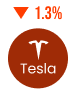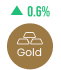# Simple Moving Averages (SMA) in Forex

• Published:
• Last Updated:

Simple moving averages (SMA) are the most basic type of moving average technical analysis indicators to use in the forex markets. It is called ‘simple’ for a few simple reasons.

It’s simple to calculate it. It’s simple to use. And most importantly, its simplicity is simply a useful trading strategy for Forex traders.

Confused? Don’t worry, we’ll make it simple.

## Calculating the Simple Moving Average (SMA)

The SMA indicator is calculated by taking a series of prices (or reporting periods), adding these together, and then dividing the total by the number of data points. For example, if you were to calculate the period SMA for ten days, you would take the recent data points of the last ten days and divide the result by ten.

Now, let’s say that the last ten data points of an asset were: 80, 81, 81, 82, 80, 82, 89, 82, 82, and 83. The moving average price would add these figures together and divide by ten, resulting in an average closing price of 82.2.

Then each time a new data point becomes available, the moving average indicator “moves” so that the average is always based only on the last same number of variables. In this case, if the next number in the sequence was 86, the oldest rate (80) would be dropped and the new average would equal 82.8.

In another example, if you plotted a 5 period simple moving average on a 1-hour chart, you would add up the historical data closing prices for the last 5 hours, and then divide that number by 5. Or if you were to plot 5 simple moving averages on a 30-minute chart, you would add up the closing prices of the last 150 minutes and then divide that number by 5.

Or if you were to plot the… Okay, okay, just kidding. I know you get it!

And to be completely honest with you, most charting packages will do all the calculations for you anyway.

But in our defense, there’s a good reason we bored you with all the math on how to calculate the simple moving average indicator on a trading chart. Understanding how an indicator works means you can adjust, tweak it and eventually create different trading strategies as the foreign exchange market environment changes. Ultimately, using the recent prices with the SMA indicator will help you figure out the overall trend of the market and find entry signals.

Soooo we take the sorry back, sorry.

### Calculating SMA in Forex Trading

Now, let’s take a look at how simple moving averages smooth out the price action in the forex market.

On the daily chart below, we will plot three different SMA’s time periods – a 10-period (short-term), a 20-period (medium-term), and a 50-period (long-term) simple moving average lines.

Let’s start off with a 10-period MA overlaid on top. Notice how the 10-period moving average seems to follow the price, but the line is smoother and lags somewhat.

Now take a look at the 20-time period MA. It is even smoother and lags even further behind the price. This isn’t particularly surprising when you consider it’s taking into account the last 20 periods rather than the last ten. For this reason, technical analysts would say that the 20-period MA technical indicator is slower (and more accurate) than the 10-period MA.

And lastly, take a look at the past performance of an asset on the 50-period MA. You can clearly see that it’s even smoother, with even more lag. At the same time, it is a slower SMA than the 20 and 10 time periods.

Of the three moving averages technical indicators, the 50 MA is the slowest while the 10 MA is the fastest. You can also see, the longer the SMA period is, the more it lags behind the price, and therefore, it has more equal weighting.

At the same time, the longer period you use for the SMA, the slower it takes to react to the price fluctuations and market news.

## Wrapping Up

Instead of just looking at the current price of the market, the MA’s allow traders to see a broader view, and provide a better understanding of the next general direction of financial instruments in the forex markets. Further, it is an excellent lagging indicator to predict trend direction and the future performance of assets. All you need to do is to learn how to insert the SMA indicator into your trading platforms and integrate it into your trading plan.

Also, to use it effectively when trading forex, most traders combine the SMA with other technical analysis indicators like support and resistance levels, exponential moving averages (EMA), RSI, etc. This could help you find key points as a buy signal and sell signal.

But just like everything else, Simple Moving Averages aren’t perfect. The problem with the simple moving average trading strategy is that they are susceptible to spikes. And when this happens, this can give us false buy and sell signals.

And nobody likes false signals…

In the next lesson, we will show you what we mean by that, and introduce you to another type of moving average indicator to avoid this problem – the exponential moving average indicator.

Your invite to our 3 day accelerator program

Build the confidence you need to become financially free.

• 20 minutes of program content per day
• Traders accreditation certificate once passed
• Strategies, daily live trading and coaching